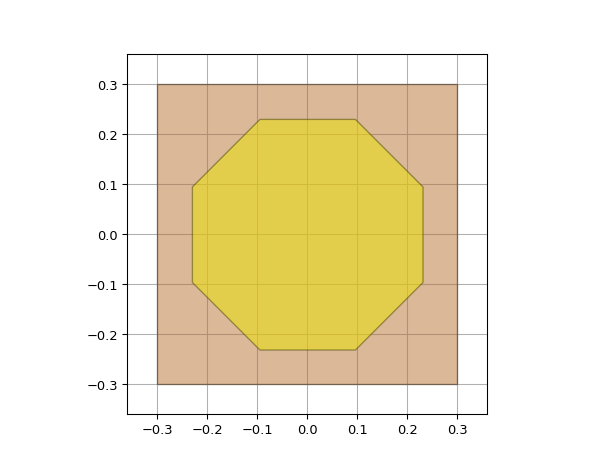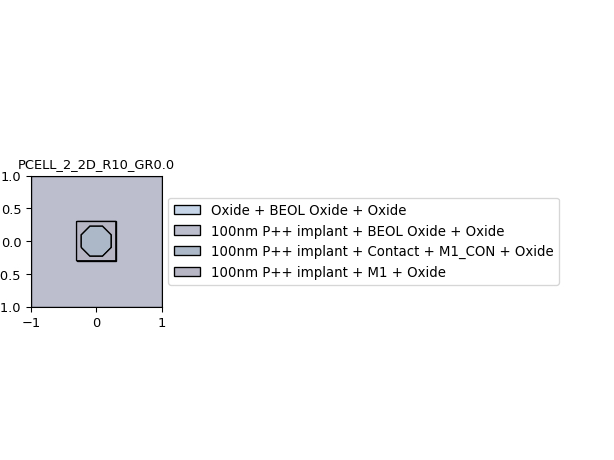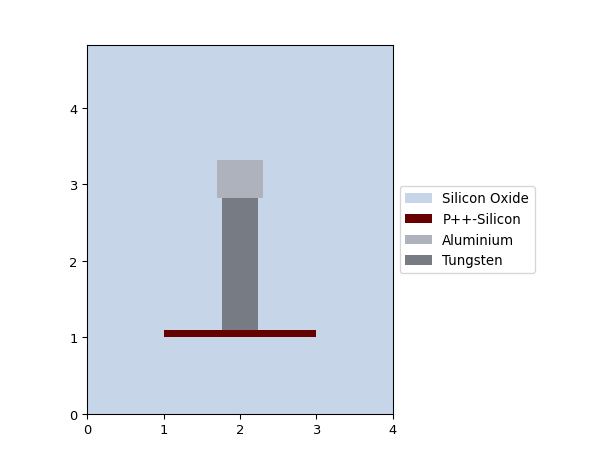# Via¶

A via is an electrical connection between two different metal layers.

## VIA_M1_M2¶

It connects M1 and M2.

from si_fab import all as pdk
from ipkiss3 import all as i3

# Via
cell = pdk.VIA_M1_M2()
lv = cell.Layout()
lv.visualize(annotate=True)
lv.visualize_2d()
xs = lv.cross_section(cross_section_path=i3.Shape([(0.0, -5.0), (0.0, 5.0)]))
xs.visualize()## Contact¶

It connects M1 with the doped regions.

from si_fab import all as pdk
from ipkiss3 import all as i3

# Contact Hole
hole = pdk.Contact()
hole_lv = hole.Layout()
hole_lv.visualize(annotate=True)

class ContactHoleWithP(i3.PCell):
class Layout(i3.LayoutView):

def _generate_instances(self, insts):
insts += i3.SRef(reference=hole)
return insts

def _generate_elements(self, elems):
elems += i3.Rectangle(box_size=(2, 2), layer=i3.TECH.PPLAYER.PPLUS)
return elems

hole_with_p_lv = ContactHoleWithP().Layout()
hole_with_p_lv.visualize_2d()
xs = hole_with_p_lv.cross_section(cross_section_path=i3.Shape([(0.0, -2.0), (0.0, 2.0)]))
xs.visualize()## HeaterContact¶

It connects M1 with the tungsten heating layer (HT).

from si_fab import all as pdk
from ipkiss3 import all as i3

# Heater contact
cell = pdk.HeaterContact()
lv = cell.Layout()
lv.visualize(annotate=True)
lv.visualize_2d()
xs = lv.cross_section(cross_section_path=i3.Shape([(0.0, -5.0), (0.0, 5.0)]))
xs.visualize()